Up: User-Defined Forces in NAMD Previous: TclBC

Subsections

Grid-steered Molecular Dynamics

In this unit, you will learn about NAMD's Grid-steered Molecular Dynamics (G-SMD) feature, also known as Gridforce. G-SMD calculates forces to be applied to target atoms based on a potential input by the user. This technique allows the fast and flexible application of spatially varying forces. Any work that uses G-SMD should cite the following paper: D.B. Wells, V. Abramkina, and A. Aksimentiev, J. Chem. Phys. 127, 125101 (2007), available at http://link.aip.org/link/?JCPSA6/127/125101/1.

Introduction

G-SMD is a method that allows one or more potentials, each defined on a grid, to be applied to a set of target atoms. Atoms are coupled to the potentials by electric charge, mass, or any other quantity, and the components of the resulting force can be scaled independently. G-SMD has many applications, including cryo-electron microscopy map fitting and acceleration of transmembrane transport.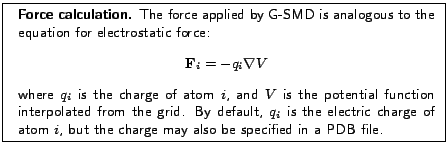Example 1: Constant Force

As a first example of G-SMD, we will apply a constant net force to a system, as we did as a first example of Tcl Forces in Section 1.

1
We first look at a simple grid for applying a constant force along the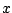direction. Go to the directory forces-tutorial-files/gridForceFiles/
push-grid and open the file constant.dx, reproduced here:

 object 1 class gridpositions counts 2 2 2 origin 0. 0. 0. delta 30. 0. 0. delta 0. 30. 0. delta 0. 0. 30. object 2 class gridconnections counts 2 2 2 object 3 class array type double rank 0 items 8 data follows 1. 1. 1. 1. 0. 0. 0. 0.

The grids used for G-SMD are given to NAMD in the form of a DX file. The first seven lines are header lines and define the geometry of the grid. Line 1 specifies that the grid isgridpoints. Line 2 specifies the origin of the grid. Lines 3-5 specify the three basis vectors of the grid. In this case, the directions of the grid basis vectors correspond to the,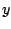, anddirections, and say that gridpoints are separated by 30 Å in each direction. Line 6 is not used by G-SMD, but the counts should be the same as those on line 1. Finally, line 7 states thatdata points compose the data.

After the header section are the values of the potential at each gridpoint. Three potential values are specified per line. The order is known asfast'', meaning that theaxis of the grid is traversed first, followed by theaxis, then theaxis. For example, the values above correspond to the following coordinates:

 (0 0 0) (0 0 1) (0 1 0) (0 1 1) (1 0 0) (1 0 1) (1 1 0) (1 1 1)

This is illustrated in Fig. 8. Thus, the grid in constant.dx has the value 1 for all gridpoints with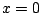, and the value 0 for all gridpoints with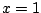, which will produce a constant force in thedirection (with certain caveats addressed below.)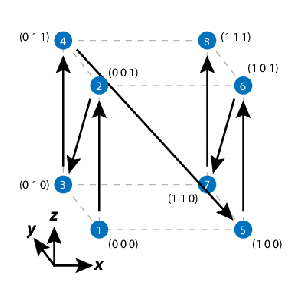2
We now make a target PDB file to tell NAMD which atoms are to be forced. Run the script make-target.tcl:

 vmd -dispdev text -e make-target.tcl

The script creates the file ubiquitin-grid.pdb. It works similarly to those you've seen previously. The beta value of protein atoms are set to 1, while the beta values of all other atoms are set to 0. In this case, since the protein is in vacuum, all atoms are given a beta value of 1. The script also sets the occupancy of the atoms to the atom's mass, which will be used as the charge'' of the atoms--by default, the electric charge of the atom is used.

3
Next, we look at the NAMD configuration file. Open the file push-grid.namd. The lines pertaining to G-SMD are listed:

 gridforce on gridforcefile ubiquitin-grid.pdb gridforcecol B gridforcechargecol O gridforcepotfile constant.dx gridforcescale 1 1 1 gridforcecont1 yes gridforcecont2 yes gridforcecont3 yes gridforcevoff -2 0 0

The keywords are described in Table 1.

Table 1: G-SMD keywords.
 Keyword Description gridforce Turns on G-SMD. gridforcevolts Whether the grid is defined in volts; default unit is kcal/molÅ. gridforcefile PDB file specifying atoms to which force will be applied. gridforcecol Which column in gridforcefile to use. The G-SMD force applied to atoms is multiplied by this value. gridforcechargecol Column from which to read atom charge; optional, default is electric charge. gridforcepotfile DX file defining grid. gridforcescale Values by which to scale the,, andcomponents of force before applying to target atoms. gridforcecont Whether the grid is continuous across periodic boundaries along this direction (see Info Box). gridforcevoff Potential offset of grid values between periodic images; only applicable along continuous directions (see Info Box).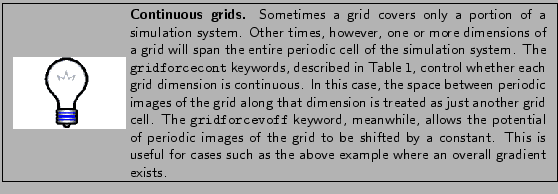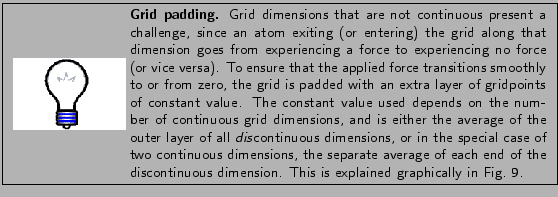4
Now run the system using NAMD:

namd2 push-grid.namd > push-grid.log

5
After NAMD finishes, we can analyze the trajectory in the same manner as for the first Tcl Forces trajectory. Run the script file plot.tcl by typing in the VMD Tk Console:

source plot.tcl

The results should be very similar to those shown in Fig. 2.

Example 2: Surface

Our next example of G-SMD is to model a surface. Specifically, we will model a graphene sheet featuring a nanogap. To make the grid, we use the VMD plugin Volmap, which can produce grids based on mass density. We then solvate and ionize the system, and apply a transverse electric field, producing an ionic current.

1
First, we load the graphene atomic structure into VMD. Navigate to the directory forces-tutorial-files/gridForceFiles/graphene, then type the following on in the Tk Console:

 mol delete all mol load psf graphene.psf pdb graphene.pdb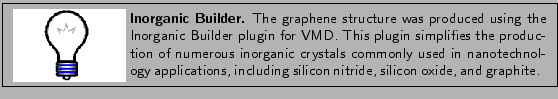2
Now run the Volmap command by typing the following in the Tk Console:

 volmap density [atomselect top all] -o graphene.dx \ -res 0.5 -minmax {{-20 -20 -5} {20 20 5}}

The command produces a density grid of all the atoms in the top molecule, with a resolution of 0.5 Å, trimmed to the range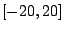inandandin.3
Next, we make the atomic system, a water box 40 Å on a side with 1.0 M NaCl. Run the following commands in the VMD Tk Console:

 solvate -o graphene-sol -minmax {{-20 -20 -20} {20 20 20}} autoionize -psf graphene-sol.psf -pdb graphene-sol.pdb \ -is 2.0 -o graphene-ion

4
As usual, we next make a target PDB file. Run the following on the command line:

vmd -dispdev text -e make-target.tcl

The resulting PDB applies G-SMD to all heavy atoms, and assigns each a grid charge of 1.

5
We now minimize the system. First examine the file graphene-min.namd. Notice that the grid is continuous in theanddirections but not thedirection. Run the minimization on the command line:

namd2 graphene-min.namd > graphene-min.log

If you watch the trajectory, you will see the water and ions expelled from the region of the graphene. This is shown in Fig. 10.6
Finally, we run the system under an external electric field equivalent to a transmembrane voltage drop of 5 V. Run the following on the command line:

namd2 graphene-elec.namd > graphene-elec.log

Example 3: Multiple grids

In this final section, we show how to use G-SMD to apply different potential grids to different sets of atoms. We will create a simulation similar to the TclBC simulation that collected all ions in a sphere, but with the twist that the different species will be placed in two different spheres. This section uses two grids, but other than memory usage, there is virtually no limit to the number of grids that can be used in a simulation.

This section also demonstrates the use of a Tcl script to write the grid file. The simplicity of the DX file format makes this a straightforward task.

1
First, we will examine the script used to write the grid files. Navigate to the directory forces-tutorial-files/gridForceFiles/ions-grid, and open the file sphere.tcl. This script constructs a DX file of a grid representing a spherically symmetric potential that is flat within a cutoff radius and increases linearly beyond it, see Fig. 12. The first few lines set the parameters of the potential:

 set gridlen 30.0 set gridnum 30 set center "0.0 0.0 10.0" set radius 5.0 set gradient 1.0 set outfile potassium.dx

The grid produced by the script, given by outfile, is a cube, with side length in angstroms given by gridlen. The number of gridpoints per side is given by gridnum. The other parameters are self-explanatory.2
Next, the script opens the output file, and writes the DX header information:

 set out [open $outfile w] puts$out "object 1 class gridpositions counts \ $gridnum$gridnum $gridnum" puts$out "origin $gridorigin" puts$out "delta $griddelta 0.0 0.0" puts$out "delta 0.0 $griddelta 0.0" puts$out "delta 0.0 0.0 $griddelta" puts$out "object 2 class gridconnections counts \ $gridnum$gridnum $gridnum" puts$out "object 3 class array type double rank 0 \ items $gridpoints data follows" 3 Finally, the script loops through the three dimensions of the grid, calculates the corresponding,, andcoordinates using the prescribed gridpoint spacing and origin, calculates the potential, and prints it to the DX file:  set n 0 for { set i 0 } {$i < $gridlen } { incr i } { set x [expr {$i * $griddelta + [lindex$gridorigin 0]}] for { set j 0 } { $j <$gridlen } { incr j } { set y [expr {$j *$griddelta + [lindex $gridorigin 1]}] for { set k 0 } {$k < $gridlen } { incr k } { set z [expr {$k * $griddelta + [lindex$gridorigin 2]}] set r [veclength [vecsub "$x$y $z"$center]] if { $r >$radius } { set v [expr {($r -$radius) * $gradient}] } else { set v 0 } puts -nonewline$out $v incr n if {$n == 3 } { puts $out "" set n 0 } else { puts -nonewline$out " " } } } } close $out The counter n is used to write three grid values per line. 4 Now run the script to produce the potential for potassium. Type the following in the VMD Tk Console: source sphere.tcl This produces the file potassium.dx. 5 Next, we make the grid for chloride ions. In sphere.tcl, change the center variable to "0.0 0.0 -10.0", and the outfile variable to chloride.dx. Then run the script again as in the previous step. 6 As usual, we must also make PDB files tagging the atoms to which G-SMD should be applied. Run the script make-target-mult.tcl: source make-target-multi.tcl The script produces two files, potassium.pdb and chloride.pdb, for the two ion species. In both files, the appropriate ions are given a charge of 1. 7 Now look at the NAMD configuration file ions-grid.namd. The keywords for G-SMD are at the end:  set scale 30 mgridforce on mgridforcefile POT potassium.pdb mgridforcecol POT B mgridforcechargecol POT O mgridforcepotfile POT potassium.dx mgridforcescale POT$scale $scale$scale mgridforcecont1 POT yes mgridforcecont2 POT yes mgridforcecont3 POT yes mgridforcefile CLA chloride.pdb mgridforcecol CLA B mgridforcechargecol CLA O mgridforcepotfile CLA chloride.dx mgridforcescale CLA $scale$scale \$scale mgridforcecont1 CLA yes mgridforcecont2 CLA yes mgridforcecont3 CLA yes

There are a few key differences that you'll notice when using multiple grids:

• First, the gridforce* keywords are replaced by the corresponding mgridforce* keywords.
• Second, all keywords except the mgridforce keyword turning on G-SMD take an extra argument identifying the grid to which the keyword applies. The identifier may be any string. In this case, the identifiers POT and CLA are used to refer to the potassium and chloride grids, respectively, but they could have been referred to as 1 and 2, A and B, aitch and seventy-two, or dave and lu--anything that describes the grid for you.
• Finally, keywords are repeated for each grid used.

8
Finally, run the simulation:

namd2 ions-grid.namd > ions-grid.log

After the simulation has completed, open the trajectory in VMD. You should see results similar to Fig. 13.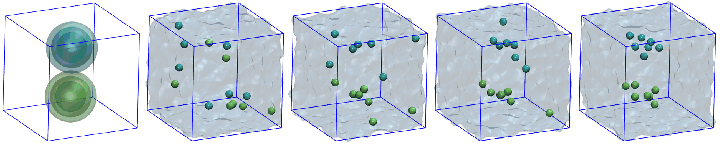Up: User-Defined Forces in NAMD Previous: TclBC
tutorial-l@ks.uiuc.edu